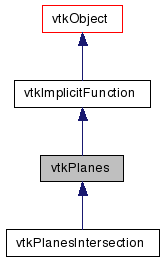# vtkPlanes Class Reference

`#include <vtkPlanes.h>`

Inheritance diagram for vtkPlanes:[legend]
Collaboration diagram for vtkPlanes:[legend]

## Detailed Description

implicit function for convex set of planes

vtkPlanes computes the implicit function and function gradient for a set of planes. The planes must define a convex space.

The function value is the closest first order distance of a point to the convex region defined by the planes. The function gradient is the plane normal at the function value. Note that the normals must point outside of the convex region. Thus, a negative function value means that a point is inside the convex region.

There are several methods to define the set of planes. The most general is to supply an instance of vtkPoints and an instance of vtkDataArray. (The points define a point on the plane, and the normals corresponding plane normals.) Two other specialized ways are to 1) supply six planes defining the view frustrum of a camera, and 2) provide a bounding box.

vtkCamera
Examples:
vtkPlanes (Examples)
Tests:
vtkPlanes (Tests)

Definition at line 52 of file vtkPlanes.h.

## Public Types

typedef vtkImplicitFunction Superclass

## Public Member Functions

virtual const char * GetClassName ()
virtual int IsA (const char *type)
void PrintSelf (ostream &os, vtkIndent indent)
void EvaluateGradient (double x, double n)
void SetFrustumPlanes (double planes)
int GetNumberOfPlanes ()
double EvaluateFunction (double x)
double EvaluateFunction (double x, double y, double z)
virtual void SetPoints (vtkPoints *)
virtual vtkPointsGetPoints ()
void SetNormals (vtkDataArray *normals)
virtual vtkDataArrayGetNormals ()
void SetBounds (double bounds)
void SetBounds (double xmin, double xmax, double ymin, double ymax, double zmin, double zmax)
vtkPlaneGetPlane (int i)
void GetPlane (int i, vtkPlane *plane)

## Static Public Member Functions

static vtkPlanesNew ()
static int IsTypeOf (const char *type)
static vtkPlanesSafeDownCast (vtkObject *o)

vtkPlanes ()
~vtkPlanes ()

## Protected Attributes

vtkPointsPoints
vtkDataArrayNormals
vtkPlanePlane

## Member Typedef Documentation

Reimplemented from vtkImplicitFunction.

Definition at line 56 of file vtkPlanes.h.

## Constructor & Destructor Documentation

 vtkPlanes::vtkPlanes ( ) ` [protected]`

 vtkPlanes::~vtkPlanes ( ) ` [protected]`

## Member Function Documentation

 static vtkPlanes* vtkPlanes::New ( ) ` [static]`

Create an object with Debug turned off, modified time initialized to zero, and reference counting on.

Reimplemented from vtkObject.

Reimplemented in vtkPlanesIntersection.

 virtual const char* vtkPlanes::GetClassName ( ) ` [virtual]`

Reimplemented from vtkImplicitFunction.

 static int vtkPlanes::IsTypeOf ( const char * name ) ` [static]`

Return 1 if this class type is the same type of (or a subclass of) the named class. Returns 0 otherwise. This method works in combination with vtkTypeMacro found in vtkSetGet.h.

Reimplemented from vtkImplicitFunction.

 virtual int vtkPlanes::IsA ( const char * name ) ` [virtual]`

Return 1 if this class is the same type of (or a subclass of) the named class. Returns 0 otherwise. This method works in combination with vtkTypeMacro found in vtkSetGet.h.

Reimplemented from vtkImplicitFunction.

 static vtkPlanes* vtkPlanes::SafeDownCast ( vtkObject * o ) ` [static]`

Reimplemented from vtkImplicitFunction.

 void vtkPlanes::PrintSelf ( ostream & os, vtkIndent indent ) ` [virtual]`

Methods invoked by print to print information about the object including superclasses. Typically not called by the user (use Print() instead) but used in the hierarchical print process to combine the output of several classes.

Reimplemented from vtkImplicitFunction.

Reimplemented in vtkPlanesIntersection.

 double vtkPlanes::EvaluateFunction ( double x ) ` [virtual]`

Evaluate plane equations. Return smallest absolute value.

Implements vtkImplicitFunction.

 double vtkPlanes::EvaluateFunction ( double x, double y, double z ) ` [inline]`

Evaluate plane equations. Return smallest absolute value.

Reimplemented from vtkImplicitFunction.

Definition at line 62 of file vtkPlanes.h.

 void vtkPlanes::EvaluateGradient ( double x, double n ) ` [virtual]`

Implements vtkImplicitFunction.

 virtual void vtkPlanes::SetPoints ( vtkPoints * ) ` [virtual]`

Specify a list of points defining points through which the planes pass.

 virtual vtkPoints* vtkPlanes::GetPoints ( ) ` [virtual]`

Specify a list of points defining points through which the planes pass.

 void vtkPlanes::SetNormals ( vtkDataArray * normals )

Specify a list of normal vectors for the planes. There is a one-to-one correspondence between plane points and plane normals.

 virtual vtkDataArray* vtkPlanes::GetNormals ( ) ` [virtual]`

Specify a list of normal vectors for the planes. There is a one-to-one correspondence between plane points and plane normals.

 void vtkPlanes::SetFrustumPlanes ( double planes )

An alternative method to specify six planes defined by the camera view frustrum. See vtkCamera::GetFrustumPlanes() documentation.

 void vtkPlanes::SetBounds ( double bounds )

An alternative method to specify six planes defined by a bounding box. The bounding box is a six-vector defined as (xmin,xmax,ymin,ymax,zmin,zmax). It defines six planes orthogonal to the x-y-z coordinate axes.

 void vtkPlanes::SetBounds ( double xmin, double xmax, double ymin, double ymax, double zmin, double zmax )

An alternative method to specify six planes defined by a bounding box. The bounding box is a six-vector defined as (xmin,xmax,ymin,ymax,zmin,zmax). It defines six planes orthogonal to the x-y-z coordinate axes.

 int vtkPlanes::GetNumberOfPlanes ( )

Return the number of planes in the set of planes.

 vtkPlane* vtkPlanes::GetPlane ( int i )

Create and return a pointer to a vtkPlane object at the ith position. Asking for a plane outside the allowable range returns NULL. This method always returns the same object. Use GetPlane(int i, vtkPlane plane) instead

 void vtkPlanes::GetPlane ( int i, vtkPlane * plane )

Create and return a pointer to a vtkPlane object at the ith position. Asking for a plane outside the allowable range returns NULL. This method always returns the same object. Use GetPlane(int i, vtkPlane plane) instead

## Member Data Documentation

 vtkPoints* vtkPlanes::Points` [protected]`

Definition at line 113 of file vtkPlanes.h.

 vtkDataArray* vtkPlanes::Normals` [protected]`

Definition at line 114 of file vtkPlanes.h.

 vtkPlane* vtkPlanes::Plane` [protected]`

Definition at line 115 of file vtkPlanes.h.

The documentation for this class was generated from the following file:

Generated on Wed Aug 24 11:55:13 2011 for VTK by1.5.6